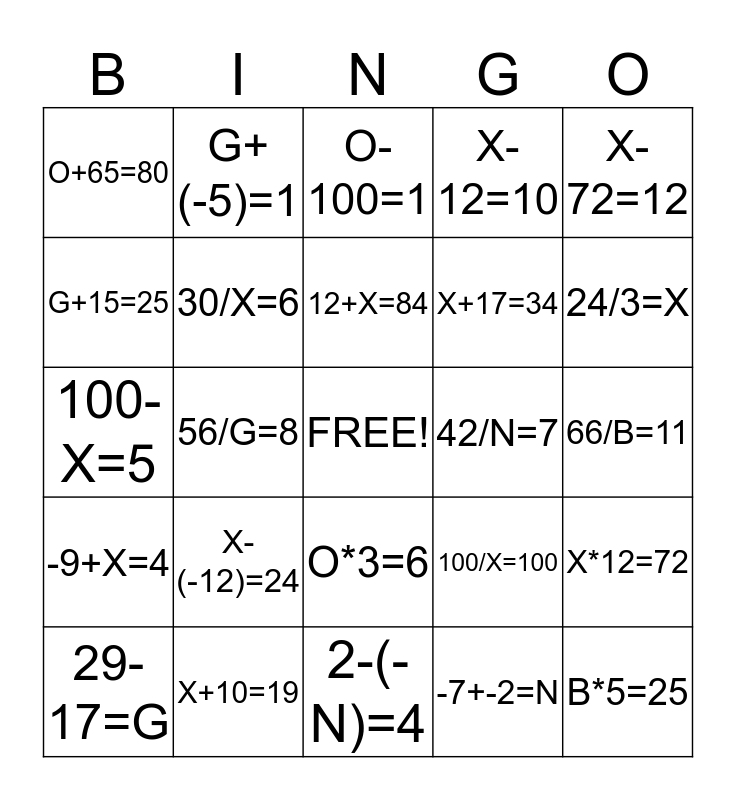# VARIABLE BingoThis bingo card has a free space and 24 words: X-(-12)=24, X+10=19, 12+X=84, 29-17=G, X-72=12, O+65=80, G+15=25, G+(-5)=1, 42/N=7, O*3=6, X+17=34, 100-X=5, 100/X=100, X*12=72, 30/X=6, -7+-2=N, 66/B=11, O-100=1, 24/3=X, 56/G=8, B*5=25, -9+X=4, X-12=10 and 2-(-N)=4.

## Play Online